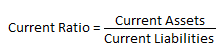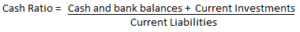## What are Liquidity Ratios: Meaning and Formulas

Financial ratio is a relationship between various items or group of items in financial statements. Financial ratios are broadly classified into five categories:
a) Liquidity Ratios
b) Leverage Ratios
c) Turnover Ratios
d) Profitability Ratios
e) Valuation Ratios

### Liquidity Ratios

Liquidity is the ability of a firm to meet its obligations in the short run, generally within one year. The important liquidity ratios are:

• Current Ratio
• Acid-test Ratio or Quick Ratio
• Cash Ratio

Current Ratio

Current Ratio is defined as ratio of Current Assets to Current Liabilities.Here

• Current Assets include cash, current investments, inventory (stock), trade receivables, loans and advances, prepaid expenses etc. These are generally converted to cash in next twelve months.
• Current Liabilities includes loans which are due to be paid in next twelve months, debtors, provisions etc.

The current ratio measures the ability of firm to meet its current liabilities. Currents assets get converted to cash during the operating cycle of firm to generate cash that is used to meet current liabilities.
The general norm for current Ratio in India is 1.33 and international standard is 2.

Acid Test Ratio or Quick Ratio

Quick Ratio is defined as ratio of Quick Assets to Current LiabilitiesHere:

• Quick assets are current assets excluding inventories.
• Current Liabilities includes loans which are due to be paid in next twelve months, debtors, provisions etc.

The quick ratio is stringent measure of liquidity. It takes into consideration only those assets which are highly liquid in nature. The stock is the least liquid among all current assets thus it is not considered while calculating the ratio.

Cash Ratio

Cash ratio is defined as Cash, bank balances and current investment to Current Liabilities.Since Cash, bank balances and short term marketable securities are most liquid assets of a firm, this ratio is most stringent measures of liquidity.

We hope you liked this article. We will discuss Leverage Ratios, Turnover Ratios, Profitability Ratios and
Valuation Ratios in another article. Here are some useful articles for you to read next:

Click to go to RBI Grade B Preparation Page

Tags: liquidity ratio, quick ratio, ratio analysis, accounting ratios, financial ratios, current ratio, current ratio formula, quick ratio formula, cash ratio, current ratio analysis, liquidity ratio analysis, liquidity ratio formula, current ratio interpretation, quick ratio interpretation, current ratio accounting, current asset ratio, acid test ratio formula, what is ratio analysis, financial ratio analysis and interpretation, what is liquidity ratio, liquidity ratio examples, liquidity ratios list.